Published: 25 April 2019

# Modal analysis of sandwich panel with composite laminated faces

Eva Kormanikova1
1Department of Structural Mechanics, Technical University of Kosice, Košice, Slovakia
Views 143

#### Abstract

A sandwich panel with laminate faces was used for free vibration analysis. The Shear Deformation Theory is considered for analysis. The effect of sandwich design parameters such as core thickness and fiber reinforced angle on vibration response is investigated. The modal analysis for various lengths of sandwich panel is done to find the limit length of the panel, which is sensitive to dynamic wind load.

#### Highlights

• A sandwich panel with laminate faces
• Free vibration analysis
• The Shear Deformation Theory
• The effect of sandwich design parameters on vibration response
• The limit length of the panel
• Sensitivity to dynamic wind load

## 1. Introduction

Sandwich panels are one of very important group of laminated composites. They consist of two thin faces with thickness of ${h}_{1}$ and ${h}_{3}$ and a core with thickness of ${h}_{2}$. The faces are made of high strength materials having good properties under tension, such as fiber reinforced polymer matrix laminates used in this paper, while the core is made of lightweight materials such as foam, having good properties under compression. Sandwich composites combine lightness and flexural stiffness .

The analysis of simple sandwich structures may be achieved by analytical methods, by adapting the classical tools of structural analysis on anisotropic elastic structure face elements. For more complex structures, such as more general boundary conditions or loading, numerical methods such as Finite Element Method, Boundary Element Method, etc. are used [2-5].

The use of assumptions is necessary to mathematical modeling of laminated composites. These include an elastic behavior of fibers and matrices, a perfect bonding between fibers and matrices, low variation of the mechanical characteristics of the individual fibers, uniform fiber diameters, their regular arrangement in the matrix, etc.

Taking into account the different size scales of mechanical modelling of structure elements composed of fiber reinforced composites, the micro, macro and structural modeling levels must be considered [6-10].

## 2. First-order shear deformation theory (FSDT)

FSDT considers that transverse normal do not remain perpendicular to the midsurface after deformation. This theory is used for thicker plates or sandwiches taking into account the Reissner kinematics (Fig. 1).

Transverse shear stresses are added to the state of plane stress for this reason. Shear deformations are written following the Fig. 2:

1
${\mathrm{\gamma }}_{xz2}=\left(\frac{{u}_{12}-{u}_{32}}{{h}_{2}}+\frac{\partial w}{\partial x}\right)=\left(\frac{{u}_{1}-{u}_{3}}{{h}_{2}}+\frac{d}{{h}_{2}}\frac{\partial w}{\partial x}\right),$
${\mathrm{\gamma }}_{yz2}=\left(\frac{{v}_{12}-{v}_{32}}{{h}_{2}}+\frac{\partial w}{\partial y}\right)=\left(\frac{{v}_{1}-{v}_{3}}{{h}_{2}}+\frac{d}{{h}_{2}}\frac{\partial w}{\partial y}\right),$

where $d$ is midplane distance of sandwich faces:

2
$d={h}_{2}+\frac{{h}_{1}+{h}_{3}}{2}.$

Fig. 1Reissner kinematics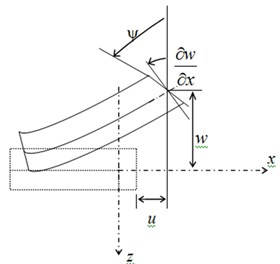a)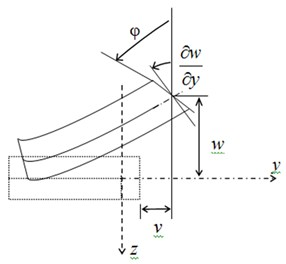b)

Fig. 2Geometry of shear deformation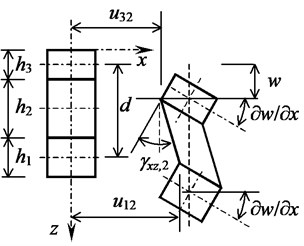a)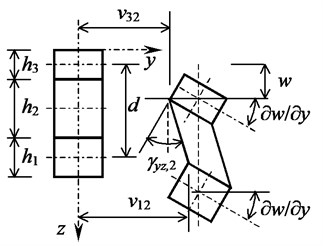b)

Internal forces are expressed by terms:

3
$\mathbf{N}=\underset{-\left(\frac{1}{2}{h}^{\left(2\right)}+{h}^{\left(1\right)}\right)}{\overset{-\frac{1}{2}{h}^{\left(2\right)}}{\int }}\mathbf{\sigma }dz+\underset{\frac{1}{2}{h}^{\left(2\right)}}{\overset{\frac{1}{2}{h}^{\left(2\right)}+{h}^{\left(3\right)}}{\int }}\mathbf{\sigma }dz,$
$\mathbf{M}=\underset{-\left(\frac{1}{2}{h}^{\left(2\right)}+{h}^{\left(1\right)}\right)}{\overset{-\frac{1}{2}{h}^{\left(2\right)}}{\int }}\mathbf{\sigma }zdz+\underset{\frac{1}{2}{h}^{\left(2\right)}}{\overset{\frac{1}{2}{h}^{\left(2\right)}+{h}^{\left(3\right)}}{\int }}\mathbf{\sigma }zdz,$
$\mathbf{V}=\underset{-\frac{1}{2}{h}^{\left(2\right)}}{\overset{\frac{1}{2}{h}^{\left(2\right)}}{\int }}\tau dz.$

The constitutive equations for a sandwich are in the form:

4
$\left(\begin{array}{c}\mathbf{N}\\ \mathbf{M}\\ \mathbf{V}\end{array}\right)=\left(\begin{array}{ccc}\mathbf{A}& \mathbf{B}& 0\\ \mathbf{C}& \mathbf{D}& 0\\ 0& 0& {\mathbf{A}}^{s}\end{array}\right)\left(\begin{array}{c}{\mathbf{\epsilon }}_{m}^{0}\\ \mathbf{\kappa }\\ \mathbf{\gamma }\end{array}\right),$

with stiffness coefficients:

5
${A}_{ij}={{A}_{ij}}^{\left(1\right)}+{{A}_{ij}}^{\left(3\right)},\mathrm{}\mathrm{}\mathrm{}{B}_{ij}=\frac{1}{2}{h}^{\left(2\right)}\left({{A}_{ij}}^{\left(3\right)}-{{A}_{ij}}^{\left(1\right)}\right),{C}_{ij}={{C}_{ij}}^{\left(1\right)}+{{C}_{ij}}^{\left(3\right)},$
${D}_{ij}=\frac{1}{2}{h}^{\left(2\right)}\left({{C}_{ij}}^{\left(3\right)}-{{C}_{ij}}^{\left(1\right)}\right),{A}_{ij}^{s}={E}_{ij}^{s}\mathrm{}{h}^{\left(2\right)},\mathrm{}\mathrm{}\mathrm{}i,j=4,5,$

where ${A}_{ij}$, ${D}_{ij}$ are coefficients of extension and bending stiffness matrix, ${B}_{ij}$, ${C}_{ij}$ are coefficients of extension-bending coupling stiffness matrix, ${E}_{ij}^{s}$ are the transverse shear moduli of the core.

## 3. Free vibrations of sandwich plates

The equations to determine the natural frequencies of the symmetric sandwich panel are used :

6
${D}_{11}\frac{{\partial }^{2}{\mathrm{\Phi }}_{x}}{\partial {x}^{2}}+{D}_{66}\frac{{\partial }^{2}{\mathrm{\Phi }}_{x}}{\partial {y}^{2}}+\left({D}_{12}+{D}_{66}\right)\frac{{\partial }^{2}{\mathrm{\Phi }}_{y}}{\partial x\partial y}-{k}^{s}{A}_{55}\left({\mathrm{\Phi }}_{x}+\frac{\partial {w}_{0}}{\partial x}\right)-{I}_{2}\frac{{\partial }^{2}{\mathrm{\Phi }}_{x}}{\partial {t}^{2}}=0,$
$\left({D}_{12}+{D}_{66}\right)\frac{{\partial }^{2}{\mathrm{\Phi }}_{x}}{\partial x\partial y}+{D}_{66}\frac{{\partial }^{2}{\mathrm{\Phi }}_{y}}{\partial {x}^{2}}+{D}_{22}\frac{{\partial }^{2}{\mathrm{\Phi }}_{y}}{\partial {y}^{2}}-{k}^{s}{A}_{44}\left({\mathrm{\Phi }}_{y}+\frac{\partial {w}_{0}}{\partial y}\right)-{I}_{2}\frac{{\partial }^{2}{\mathrm{\Phi }}_{y}}{\partial {t}^{2}}=0,$
${k}^{s}{A}_{55}\left(\frac{\partial {\mathrm{\Phi }}_{x}}{\partial x}+\frac{{\partial }^{2}w}{\partial {x}^{2}}\right)+{k}^{s}{A}_{44}\left(\frac{\partial {\mathrm{\Phi }}_{y}}{\partial y}+\frac{{\partial }^{2}{w}_{0}}{\partial {y}^{2}}\right)-{\rho }_{m}h\frac{{\partial }^{2}{w}_{0}}{\partial {t}^{2}}=0,$

where ${k}^{s}$ is the transverse shear deformation factor given by value 5/6:

7
${\rho }_{m}=\frac{1}{h}\sum _{k=1}^{N}{\rho }_{k}\left({z}^{\left(k\right)}-{z}^{\left(k-1\right)}\right),\mathrm{}\mathrm{}\mathrm{}I=\frac{{\rho }_{m}{h}^{3}}{12}\frac{1}{3}\sum _{k=1}^{N}{\rho }_{k}\left({z}^{\left(k\right)}{\right)}^{3}-\left({z}^{\left(k-1\right)}{\right)}^{3},$

where ${\rho }_{k}$ is the mass density of the $k$th layer.

For the simply supported plate let:

8
${w}_{0}\left(x,y,t\right)={\mathrm{w}}_{0}^{°}{e}^{i{\omega }_{mn}t},\mathrm{}\mathrm{}\mathrm{}{\mathrm{\Phi }}_{x}\left(x,y,t\right)={\mathrm{\Phi }}_{x}^{°}{e}^{i{\omega }_{mn}t},\mathrm{}\mathrm{}\mathrm{}{\mathrm{\Phi }}_{y}\left(x,y,t\right)={\mathrm{\Phi }}_{y}^{°}{e}^{i{\omega }_{mn}t},$

where $m$, $n$ – are integers only, ${\omega }_{mn}$ – is natural angular velocity.

Ultimate length of sandwich panel depends on the ultimate frequency ${f}_{0,lim}^{}$ of dynamic loading affected to the structures:

9
${L}_{0,lim}=\sqrt{\frac{\pi {m}^{2}\left(\sqrt{{k}_{s}^{2}{A}_{{}_{55}}^{2}{D}_{11}h{\rho }_{m}+{\pi }^{2}{D}_{11}{h}_{{}_{}}^{2}{\rho }_{{}_{m}}^{2}{f}_{0,lim}^{2}}-\pi {D}_{11}h{\rho }_{m}{f}_{0,lim}^{}\right)}{2{k}_{s}^{}{A}_{{}_{55}}^{}{h}_{}{\rho }_{m}{f}_{0,lim}^{}}}.$

## 4. Numerical solution of sandwich panels

For the numerical solution, the simply supported panel with laminate facings was used. Panel length is 3250, 4250, 5250, 6250, 7100 mm, nominal width is 1000 mm. The thickness of the panel is 40, 80 and 120 mm. The thickness of laminate facings is 1 mm, composed of eight layers of symmetric [0/±45/90]s, [0/90]2s and [0/0]2s laminates, respectively. The thickness of each laminate layer is 0.125 mm. Carbon fibers in epoxy matrix were considered , with the following characteristics: ${E}_{f}=$ 230 GPa; ${E}_{m}=$ 3 GPa; ${\nu }_{f}=$0.3; ${\nu }_{m}=$ 0.3; $\xi =$ 0.6; ${\rho }_{c}=$ 1508 kg/m3. Sandwich core, consisting of PUR foam, has material constants: ${E}_{PUR}=$ 25 MPa; ${\nu }_{PUR}=$ 0.3; ${\rho }_{PUR}=$ 100 kg/m3.

Firstly, it has been done the modal analysis of the sandwich panels with a thickness of 40, 80 and , composed of eight layers of symmetric [0/±45/90]s, [0/90]2s and zero angles [0/0]2s laminates as sandwich outer layers for 3250 mm panel length.

Table 1 shows the first natural frequency depending on the variation of fiber reinforced angle of the laminate facings and the core thickness of the sandwich panel. The natural frequencies depend on the rotation of individual layers to the global coordinate system, while the lowest value of first natural frequency was obtained for the sandwich panel with [0/45/-45/90]s laminate faces and panel thickness 40 mm. Dependence of the natural frequencies from its natural mode shape of vibration by various faces is shown in the Fig. 3.

Table 1First natural frequencies f [Hz] of sandwich panels with length L= 3250 mm

 Natural mode shape Laminate [0/90]2s Laminate [0/±45/90]s Laminate [0/0]2s Thickness [mm] Thickness [mm] Thickness [mm] 40 80 120 40 80 120 40 80 120 1 13.7 21.8 27.7 11.9 18.5 23.6 18.5 29.2 36.7

Secondly, the modal analysis for various lengths of the sandwich panel with [0/45/-45/90]s laminate faces was made to find the limit length of the panel, which is sensitive to dynamic wind load with ${f}_{0,lim}=$ 5 Hz. The limit length of sandwich panels with [0/45/-45/90]s laminate faces was found for various thicknesses of the sandwich. For sandwich panel 40, 80 and 120 mm thick, the limit length is 5.0 m, 6.25 m and 7.1 m, respectively. These types of sandwich panels are not sensitive to dynamic wind load under calculated limit lengths (Fig. 4).

Fig. 3Natural frequencies [Hz] of the first ten natural mode shapes of sandwich panels with 80 mm thickness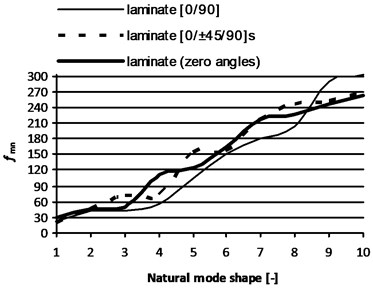Fig. 4First natural frequencies [Hz] of sandwich panels with symmetric [0/±45/90]s laminate facings versus sandwich length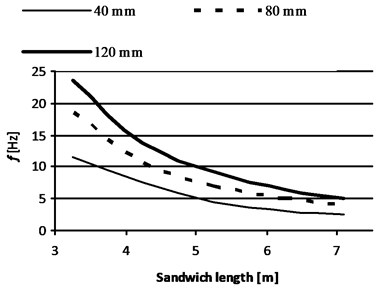## 5. Conclusions

The mechanical properties of sandwich panels with laminate faces were investigated. Computational program MATLAB was used to calculate the effective material properties of laminate faces [12, 13]. The type of faces of a sandwich panel and the core thickness affect its natural frequencies. The first natural frequency of investigated laminate panels with the length $L=$ 3250 mm is up to ${f}_{0,lim}=$ 5 Hz (Table 1), therefore the panels are not sensitive to the dynamic wind load. The sandwich panels with [0/45/-45/90]s laminate faces and 40, 80 and 120 mm thick are not sensitive to dynamic wind load under their ultimate length 5.05 m, 6.25 and 7.1 m, respectively (Fig. 4).

#### Cited by

Multi-objective optimization of composite sandwich panels using lamination parameters and spectral Chebyshev method
Passive Multi-Layer Composite Damper of Flat Belt Tensioner Idler

15 March 2019
Accepted
22 March 2019
Published
25 April 2019
SUBJECTS
Modal analysis and applications
Keywords
sandwich panel
mode shape
natural frequencies
limit length
Acknowledgements

This work was supported by the Scientific Grant Agency of the Ministry of Education of Slovak Republic and the Slovak Academy of Sciences under Projects VEGA 1/0374/19 and 1/0078/16.